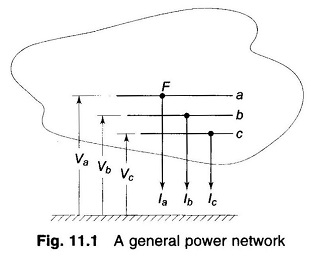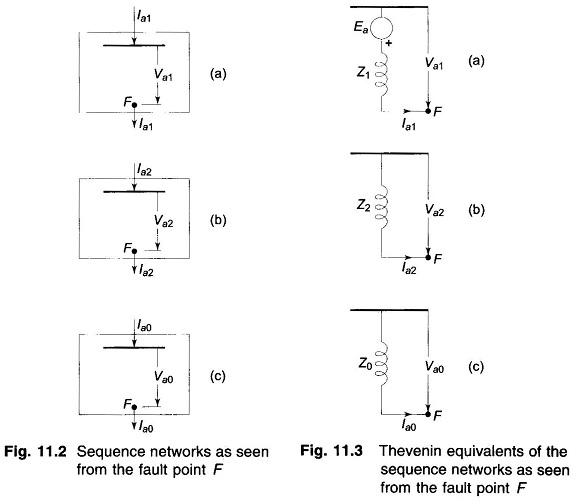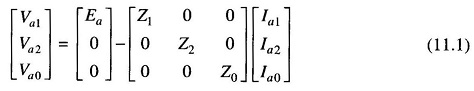## Symmetrical Component Analysis of Unsymmetrical Faults:

Symmetrical Component Analysis of Unsymmetrical Faults – Consider a general power network shown in Fig. 11.1. It is assumed that a shunt type fault occurs at point F in the system, as a result of which currents Ia, Ib, Ic flow out of the system, and Va, Vb, Vc are voltages of lines a, b, c with respect to ground.Let us also assume that the system is operating at no load before the occurrence of a fault. Therefore, the positive sequence voltages of all synchronous machines will be identical and will equal the prefault voltage at F. Let this voltage be labelled as Ea.As seen from F, the power system will present positive, negative and zero sequence networks, which are schematically represented by Figs. 11.2a, b and c. The reference bus is indicated by a thick line and the point F is identified on each sequence network. Sequence voltages at F and sequence currents flowing out of the networks at F are also shown on the sequence networks. Figures 11.3a, b, and c respectively, give the Thevenin equivalents of the three sequence networks.

Recognizing that voltage Ea is present only in the positive sequence network and that there is no coupling between sequence networks, the sequence voltages at F can be expressed in terms of sequence currents and Thevenin sequence impedances asDepending upon the type of fault, the sequence currents and voltages are constrained, leading to a particular connection of sequence, networks. The sequence currents and voltages and fault currents and voltages can then be easily computed. We shall now consider the various types of faults enumerated earlier.